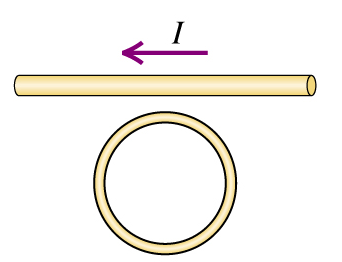# Problem: The current in the figure (Figure 1) obeys the equation I(t) = I0e-bt, where b &gt; 0. Find the direction (clockwise or counterclockwise) of the current induced in the round coil for t &gt; 0.

###### FREE Expert Solution

The induced current in the coil:

$\overline{){\mathbf{I}}{\mathbf{=}}{{\mathbf{I}}}_{{\mathbf{0}}}{{\mathbf{e}}}^{\mathbf{-}\mathbf{b}\mathbf{t}}}$, b > 0

According to Lenz's law, an induced current or emf always opposes the change in the magnetic field that causes it.

The current is passing through a straight line creating a magnetic field around it.

The current through the straight line is proportional to the magnitude of the magnetic field.

98% (450 ratings)###### Problem Details

The current in the figure (Figure 1) obeys the equation I(t) = I0e-bt, where b > 0.Find the direction (clockwise or counterclockwise) of the current induced in the round coil for t > 0.